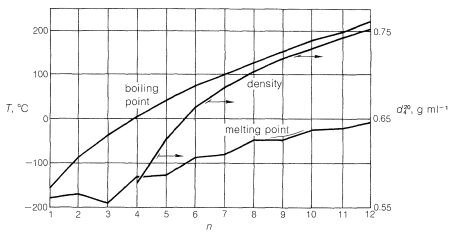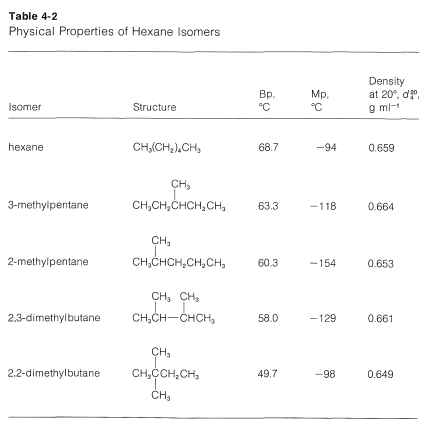# 4.2: Physical Properties of Alkanes and The Concept of Homology

$$\newcommand{\vecs}{\overset { \rightharpoonup} {\mathbf{#1}} }$$ $$\newcommand{\vecd}{\overset{-\!-\!\rightharpoonup}{\vphantom{a}\smash {#1}}}$$$$\newcommand{\id}{\mathrm{id}}$$ $$\newcommand{\Span}{\mathrm{span}}$$ $$\newcommand{\kernel}{\mathrm{null}\,}$$ $$\newcommand{\range}{\mathrm{range}\,}$$ $$\newcommand{\RealPart}{\mathrm{Re}}$$ $$\newcommand{\ImaginaryPart}{\mathrm{Im}}$$ $$\newcommand{\Argument}{\mathrm{Arg}}$$ $$\newcommand{\norm}{\| #1 \|}$$ $$\newcommand{\inner}{\langle #1, #2 \rangle}$$ $$\newcommand{\Span}{\mathrm{span}}$$ $$\newcommand{\id}{\mathrm{id}}$$ $$\newcommand{\Span}{\mathrm{span}}$$ $$\newcommand{\kernel}{\mathrm{null}\,}$$ $$\newcommand{\range}{\mathrm{range}\,}$$ $$\newcommand{\RealPart}{\mathrm{Re}}$$ $$\newcommand{\ImaginaryPart}{\mathrm{Im}}$$ $$\newcommand{\Argument}{\mathrm{Arg}}$$ $$\newcommand{\norm}{\| #1 \|}$$ $$\newcommand{\inner}{\langle #1, #2 \rangle}$$ $$\newcommand{\Span}{\mathrm{span}}$$$$\newcommand{\AA}{\unicode[.8,0]{x212B}}$$

The series of straight-chain alkanes, in which $$n$$ is the number of carbons in the chain, shows a remarkably smooth gradation of physical properties (see Table 4-1 and Figure 4-1). As $$n$$ increases, each additional $$CH_2$$ group contributes a fairly constant increment to the boiling point and density, and to a lesser extent to the melting point. This makes it possible to estimate the properties of an unknown member of the series from those of its neighbors. For example, the boiling points of hexane and heptane are $$69^\text{o}$$ and $$98^\text{o}$$, respectively. Thus a difference in structure of one $$CH_2$$ group for these compounds makes a difference in boiling point of $$29^\text{o}$$; we would predict the boiling point of the next higher member, octane, to be $$98^\text{o} + 29^\text{o} = 127^\text{o}$$, which is close to the actual boiling point of $$126^\text{o}$$.Figure 4-1: Dependence on $$n$$ of melting points, boiling points, and densities ($$d_4^{20}$$) of continuous-chain alkanes, $$CH_3 \left( CH_2 \right)_{n-1}H$$

Members of a group of compounds, such as the alkanes, that have similar chemical structures and graded physical properties, and which differ from one another by the number of atoms in the structural backbone, are said to constitute a homologous series. When used to forecast the properties of unknown members of the series, the concept of homology works most satisfactorily for the higher-molecular-weight members because the introduction of additional $$CH_2$$ groups makes a smaller relative change in the overall composition of such molecules. This is better seen from Figure 4-2, which showsFigure 4-2: Dependence of $$\Delta T$$ (difference in boiling and melting points between consecutive members of the series of continuous-chain alkanes) on $$n$$ (number of carbon atoms)

how $$\Delta T$$, the differences in boiling points and melting points between consecutive members of the homologous series of continuous-chain alkanes, changes with the number of carbons, $$n$$.

Branched-chain alkanes do not exhibit the same smooth gradation of physical properties as do the continuous-chain alkanes. Usually there is too great a variation in molecular structure for regularities to be apparent. Nevertheless, in any one set of isomeric hydrocarbons, volatility increases with increased branching. This can be seen from the data in Table 4-2, which lists the physical properties of the five hexane isomers. The most striking feature of the data is the $$19^\text{o}$$ difference between the boiling points of hexane and 2,2-dimethylbutane.Figure 4-1 for alkanes. This generally will be true, except when the number of carbons is small and when the hydrocarbon chain has polar substituents. To explain briefly, consider compounds such as alcohols, $$ROH$$, which have polar $$\overset{\delta \ominus}{O}$$- - -$$\overset{\delta \oplus}{H}$$ groups. As we indicated in Section 1-3, polarity causes molecules to associate with one another, which decreases their volatility, raises melting points, increases solubility in polar liquids, and decreases solubility in nonpolar liquids. This explains why methanol, $$CH_3OH$$, is much less volatile and much more water-soluble than methane, $$CH_4$$. But we find that the water-solubility of alcohols falls off rapidly with the length of the carbon chain, certainly faster than expected for a simple homologous series effect. Whereas methanol, $$CH_3OH$$, and ethanol, $$CH_3CH_2OH$$, are completely soluble in water, butanol, $$CH_3CH_2CH_2CH_2OH$$, is only slightly soluble. This illustrates the conflicting properties conferred on molecules by polar groups compare to nonpolar hydrocarbon groups, and points up that large changes in physical properties can be expected in the early part of a homologous series until the hydrocarbon chain is sufficiently long, usually six or more carbons, so that the hydrocarbon parts dominate over the polar parts of the molecules.